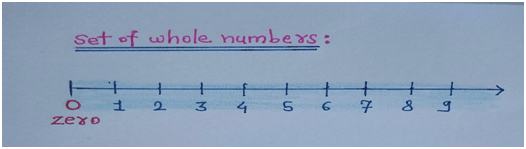# Whole numbers

Without numbers we can’t do anything, wherever we will go there will be the things related to numbers and counting.In mathematics without numbers nothing is possible, numbers are the backbone of maths.

For example:

• Counting money, days, months and all the things are counted using numbers only.
• Numbers are playing most important role in mathematics.
• There are various kinds of numbers like prime, composite, even, odd, natural, whole, rational, irrational, decimal etc.

### Whole numbers and other sets of numbers:

• Whole numbers are the real numbers which are starting from 0, 1, 2, 3, 4, 5…..up to infinity.
• The numbers like ½, 6.7 or 88.9 does not includes in the set of whole numbers.• The important thing is that zero is included in the set of whole numbers.
• The set of whole number is denoted by W.
• As whole numbers are 0,1,2,3,4,…. and so on, we can say that whole numbers includes positive integers with zero.
• And also we say that all the whole numbers are natural numbers.
• The set of whole number does not include negative integers, fractions, and decimals so its name is whole number.
• Zero is the smallest and first whole number from the set of whole numbers.
• Also, set of natural numbers N={1,2,3,4,5,….} is the subset of whole numbers.• The difference between whole numbers and natural numbers is whole numbers includes zero while natural numbers does not include zero.
• The counting numbers also starts from 1, 2, 3, 4, …. And so on.• Thus, set of whole number W, set of natural number N are the proper subsets of set of integers.
• And the set of natural number is the proper subset of set of whole numbers as shown in figure below.• The set of integers I={……-4,-3,-2,-1,0,1,2,3,4…} contains set of whole numbers also. Hence, set of whole numbers is the proper subset of integers.• Hence, we conclude that every natural number is a whole number.
• And except zero, every whole number is the natural number.

### Properties of whole numbers

There are some properties of whole numbers which are explained as follows.

1) Closure property

2) Associative property

3) Commutative property

4) Distributive property

1) Closure property

• If we add any two whole numbers then their sum is also the whole number.
• For example: 3&6 are the whole numbers then their addition 3+6=9, 9 is also whole number.
• Hence, closure property over addition is satisfied by set of whole numbers.

• Again, if we take subtraction of any two whole numbers then the answer we got is also the whole number.
• For example: 8&2 are the whole numbers, then 8 – 2=6 and 6 is also the whole number.
• Hence, closure property over subtraction is also satisfied by set of whole numbers.

• Also, if we multiply any two whole numbers then their multiplication is also the whole number.
• For example: 4&3 are the whole numbers, then 4*3= 12 and 12 is also the whole number.
• Hence the closure property over multiplication is also satisfied by the set of whole numbers.

2) Associative property:

The addition and multiplication is associative in case of set of whole numbers.

For example:

2,3&4 are the whole numbers then,

2+(3+4)=(2+3)+4

And 2*(3*4)=(2*3)*4

Hence, associative property over addition and multiplication is satisfied by set of whole numbers.

3) Commutative property:

If we reversed the order of whole numbers in case of addition and multiplication then the answer will not be changed.

For example:

4 & 5 are the whole numbers, then

4+5= 5+ 4 and 4*5= 5*4

Hence, we can say that commutative property over addition and multiplication is satisfied by the set of whole numbers.

4) Distributive property:

According to this property, the whole numbers are distributed over addition.

For example:

If we have taken the whole numbers as 2,3&4 then

2*(3+4)=2*3+2*4

Hence, we can say that whole numbers are distributive over addition.

As set of whole numbers includes zero, hence zero is the additive identity because if in zero we add any whole number then we will get the same number.

For example:

5 &0 are the whole numbers then,

5 + 0 = 0 + 5 = 5

Hence, zero acts as additive identity in case of set of whole numbers.

Multiplicative identity:

The set of whole numbers includes one, and if we multiply any whole number by one then we will get the same whole number.

For example:

6 & 1 are the whole numbers then,

6*1 = 1*6 = 6

Hence, in a set of whole numbers one acts as multiplicative identity.

Number Names MCQ

Number System MCQ

Ordinal Numbers MCQ

Consecutive Numbers MCQ

Integers MCQ

Natural Numbers MCQ

Whole Numbers MCQ

Even Numbers MCQ

Updated: November 3, 2022 — 2:49 pm